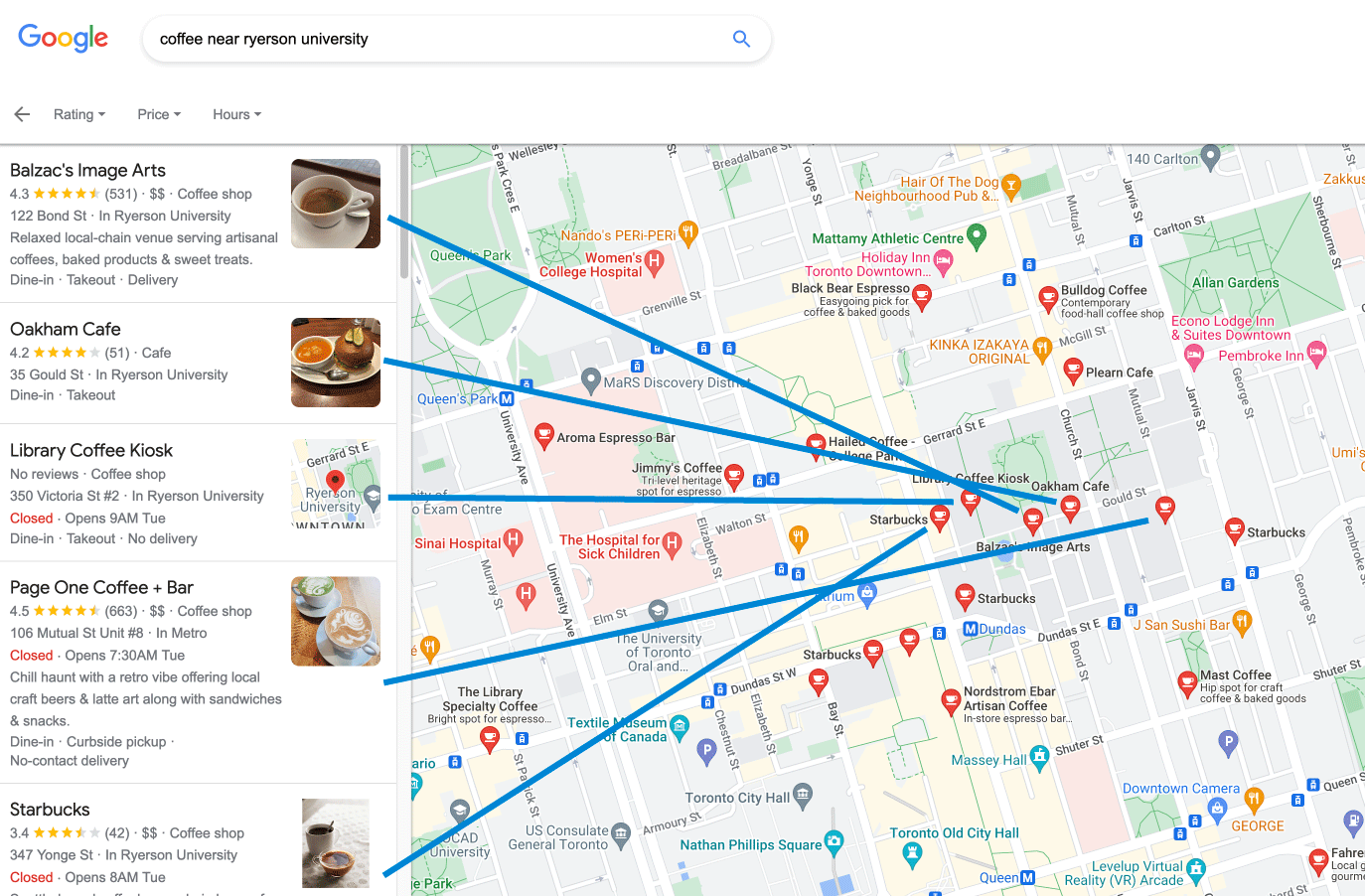Suppose you have a map, with certain points of interest marked. To avoid cluttering the map with the labels of these points, you want to list the labels in a line down the side of the map, with each point of interest connected to its label by a line segment. Preferably, the line segments should not cross each other, as they did when I tried to match the sidebar to the points in this example from Google Maps:One way to find a non-crossing labeling (for points with distinct $$y$$-coordinates) would be to make all of the line segments horizontal, and to sort the list of labels by the height of the points. However, that’s far from the only possibility. How many different permutations of the labels can a single set of points have?

The obvious bound is factorial, but the answer turns out to be much smaller than that. To see this, assume without loss of generality that the points are in general position (collinearities or equal coordinates can only reduce the number of labeling orders). Consider any non-crossing labeling, and then adjust the right endpoint of each line segment (the one on the point of interest) to have the same $$y$$-coordinate as its left endpoint, so that all of the line segments become straight.Next, connect the right endpoints of this diagram in a Cartesian tree. This is a non-crossing binary tree, rooted at the rightmost endpoint, with two subtrees constructed recursively in the same way for the parts of the diagram above and below the root segment.The number of possible binary trees is a Catalan number, and each binary tree uniquely determines a labeling order. To reconstruct the labeling from the tree, count the number of segments that lie above the root segment in the tree, and draw a line segment connecting the corresponding point of interest to the sidebar line, splitting the remaining points of interest into the corresponding number of points above and below the segment. Combinatorially, there is only one way of making a split that subdivides the remaining points into subsets of the desired sizes. Then continue recursively in the same way within the two subtrees above and below the root segment. You may need to adjust the geometric positions of the left endpoints of some of the line segments to avoid crossings, and this adjustment may not be possible for some binary trees, but when it is possible there is only one permutation of the points that you can get for each tree. Therefore, there is at most a Catalan number of labeling orders!

The same argument also works to count labelings with L-shaped connectors, horizontal from the label to the $$x$$-coordinate of the point of interest and then vertical up or down to the point of interest. When the points of interest all have distinct coordinates, no adjustment of positions is ever necessary, and every binary tree produces a valid labeling order, so the number of labeling orders is exactly a Catalan number.

For some sets of points with straight line segment labelings, you can again get the maximum possible Catalan number of distinct labeling orders. This is true, for instance, when each point of interest is to the right of all of the lines through pairs of points to the left of it (including candidate left endpoints of connectors), because in this case the combinatorics of straight line connectors and L-shaped connectors are the same. (For details of this equivalence, see my book Forbidden Configurations in Discrete Geometry, Chapter 14: “The Stretched Geometry of Permutations”.)

(Discuss on Mastodon)